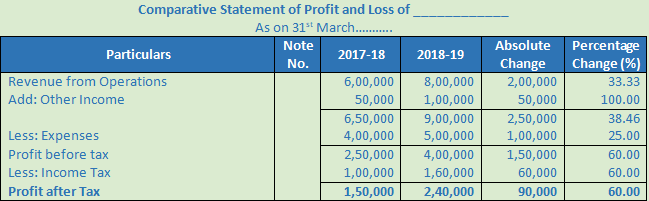# DK Goel Solutions Class 12 Accountancy Chapter 3 Tools for Financial Analysis

Read DK Goel Class 12 Accountancy Solutions for Chapter 3 Tools for Financial Analysis below. These DK Goel Accountancy Class 12 solutions have been prepared based on the latest book for DK Goel Class 12 for the current academic year by expert accounts teachers at studiestoday.com. These DK Goel Class 12 Solutions help commerce students in class 12 understand accountancy and build a strong base in accounts. Students in Class 12 who study accountancy and use the DK Goel Accountancy book to understand concepts of Chapter 3 Tools for Financial Analysis should understand the concepts and solve practice questions and exercises given at the end of the chapter. We have provided solutions for all questions and have also provided short notes for each problem. This will help Class 12 DK Goel Accountancy students to understand the questions properly. Refer to the solutions provided below prepared by CBSE NCERT teachers

## Chapter 3 Tools for Financial Analysis DK Goel Class 12 Solutions

Class 12 Accountancy students should read the following DK Goel Solutions for Class 12 Chapter 3 Tools for Financial Analysis in Standard 12. All solutions provided below can be downloaded in Pdf and are available for free. This DK Goel Book for Grade 12 Accountancy will be very useful for exams and help you to score good marks in Class 12 accountancy examinations. On our website www.studiestoday.com, we have provided solutions for all chapters given in the DK Goel Accountancy Book for Class 12.

### DK Goel Solutions Chapter 3 Tools for Financial Analysis Class 12 Accountancy

Question 1. What is meant by Comparative Financial Statements? Explain their objectives.

Solution 1. When financial statements figures for two or more years are placed side-by-side to facilitate comparison, these are called ‘Comparative Financial Statements’. In such a statement figures of production, revenue from operations, expenses, profits etc. are put side-by-side to draw conclusions about the profitability and financial health of the business. It also indicates the trend of change as well as the strong points and weak points of the enterprise.

Question 2. What is Comparative Balance Sheet? How is it prepared?

Solution 2. A single year’s balance sheet shows only the balance of accounts on a particular date, whereas the comparative balance sheet shows not only the balances of accounts as on different dates but also the extent of increase or decrease in various items of balance sheet.

The form of comparative balance sheet consists of five columns. In the first column the items of Balance Sheet are entered. In the second column the date for previous year is shown and in the third column the date for current year is shown. In the fourth column the increases or decreases in absolute data is shown in terms of rupee amounts. Fifth column shows the percentage of increase or decrease in absolute data.

Question 3. What is Comparative Statement of Profit and Loss? How is it prepared?

Solution 3. Statement of Profit and Loss shows the net profit or net loss of that year. A comparative statement of profit and loss shows the net profits for a number of years so that changes in absolute data in term of money as well as in terms of percentages may be known. Comparative statement of profit and loss provides the following information’s:

i. Rate of increase or decrease in revenue from operations.

ii. Rate of increase or decrease in cost of materials consumed.

iii. Rate of increase or decrease in incomes and expenses.

iv. Rate of increase of decrease in net profit.

The form of comparative statement of profit and loss also consists of five columns. In the first column items of statement of Profit & Loss are shown. In the second column the data for previous year is shown and in the third column the date for current year is shown. In the fourth column the increase or decrease in absolute data is shown in term of rupee amounts.

Question 4. Give a specimen of Comparative Balance Sheet of a Company for two years for comparison purpose.

Solution 4.Question 5. Give a specimen of Comparative Statements of Profit & Loss for two years.

Solution 5Question 6. Take a few imaginary figures and prepare a Comparative Statement of Profit & Loss for two years.

Solution 6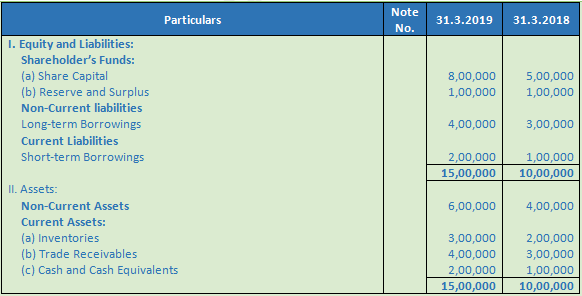Numerical Questions

Question 1. From the following Balance Sheets of Royal Industries as at 31st March, 2018 and 2019, prepare a comparative Balance Sheet: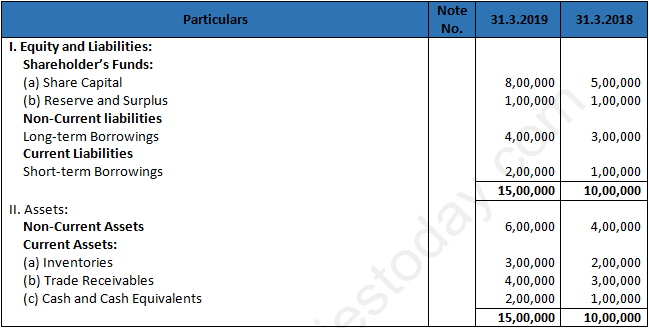Solution 1.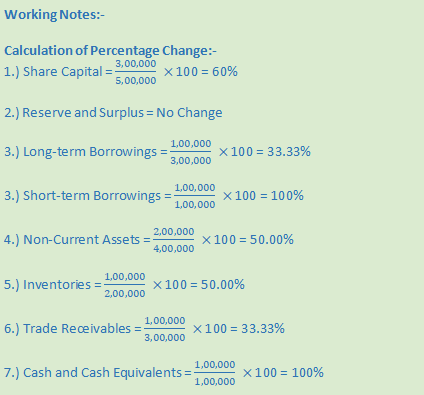Question 2. From the following information, prepare Comparative Balance Sheet of Y Ltd :Solution 2.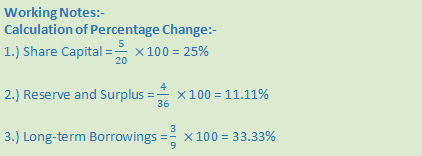Question 3. Prepare a Comparative Balance Sheet from the following and interpret it.: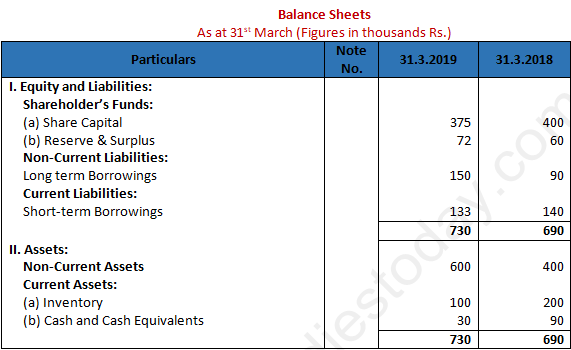Solution 3.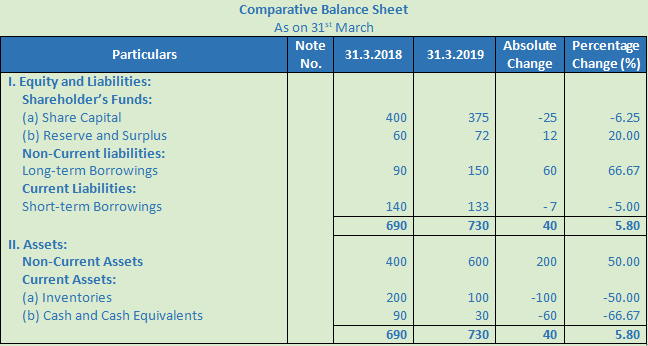Question 4. Prepare a comparative statement of Profit & Loss from the following data: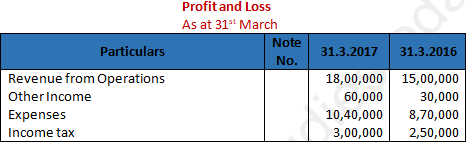Solution 4.Question 5. Prepare a Comparative Statement of Profit & Loss from the following:-Interest on investments @ Rs. 50,000 each year.

Solution 5.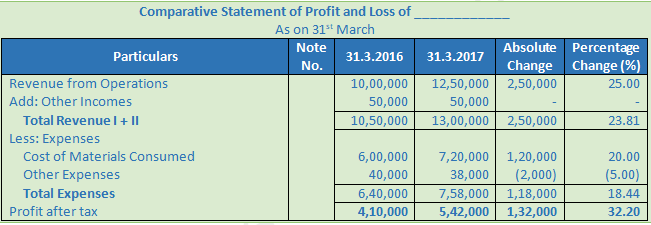Question 6. With the help of the following information prepare a Comparative Statement of Profit & Loss of Gopal Ltd.Solution 6.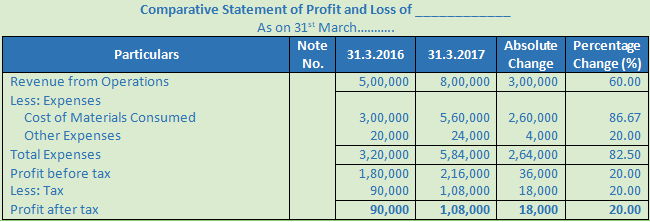Question 7. From the following information, prepare a Comparative Statement of Profit & Loss: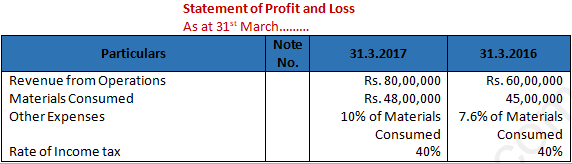Solution 7.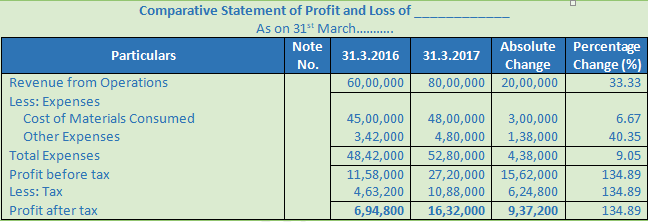Question 8. From the following Statement of profit and loss of the Sakho Ltd. for the years ended 31st March 2015 and 2016, prepare Comparative Statement of Profit & Loss.Solution 8.Question 9. Prepare a ‘Comparative Statement of Profit and Loss’ from the following information:Solution 9.Question 10. From the following information provided, prepare Comparative Statement of Profit and Loss for the year ended 31st March 2018 and 2017:Solution 10.Question 11. From the following information, prepare Comparative Statement of Profit and Loss:Solution 11.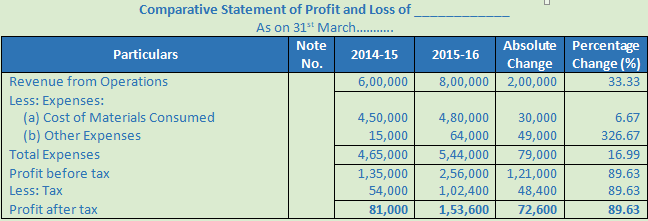Working Note:-

Calculation of Other Expenses:-

Gross Profit = Revenue from Operations – Cost of Materials Consumed

For the Year of 2014-15

Gross Profit = Rs. 6,00,000 – Rs. 4,50,000

Gross Profit = Rs. 1,50,000

For the Year of 2015-16

Gross Profit = Rs. 8,00,000 – Rs. 4,80,000

Gross Profit = Rs. 3,20,000

Other Expenses (2014-15) = Rs. 1,50,000 × 10% = Rs. 15,000

Other Expenses (2015-16) = Rs. 3,20,000 × 20% = Rs. 64,000

Question 12. Prepare a Comparative Statement of Profit and Loss with the help of the following information:Solution 12.Question 13. Prepare a Comparative Statement of Profit and Loss from the following information: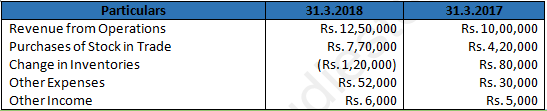Solution 13.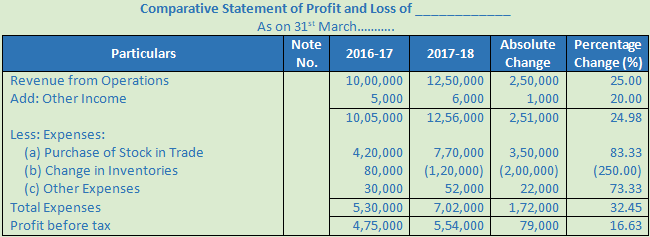Question 14. Prepare a Comparative Statement of Profit and Loss from the following information: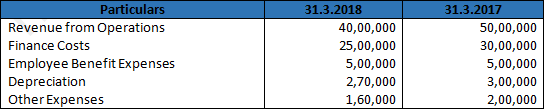Solution 14.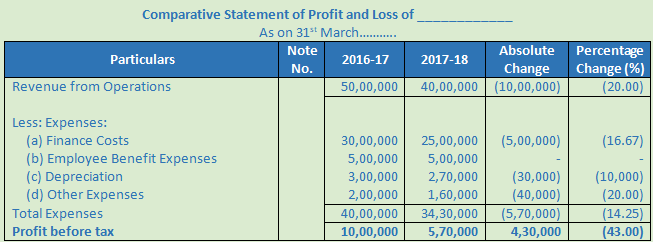Question 15. Prepare a Comparative Statement of Profit and Loss from the following:Solution 15.Question 16. The motto of Yash Ltd. and advertising company is ‘Service with Dignity’. Its management and work force is hard-working, honest and motivated. The net profit of the company doubles during the year ended 31.03,2014. Encourage by its performance company decided to give one month extra salary to all employees. Following is the Comparative Statement of Profit and loss of the company for the year ended 31st March 2013 and 2014.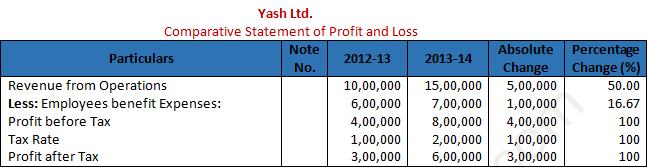(a) Calculate Net Profit Ratio for the years ending 31st March, 2013 and 2014.

(b) Identify any two values which Yash Ltd. is trying to propagate.

Solution 16.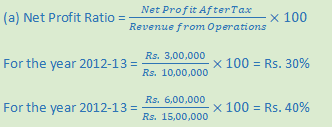(b) Values which Yash Ltd. is trying to propagate:-

(i) Recognition of hardwork and honesty of employees.

(ii) Ethical practices of Company.

Question 17. From the following information, prepare a Comparative Balance Sheet of Depth Ltd.Solution 17.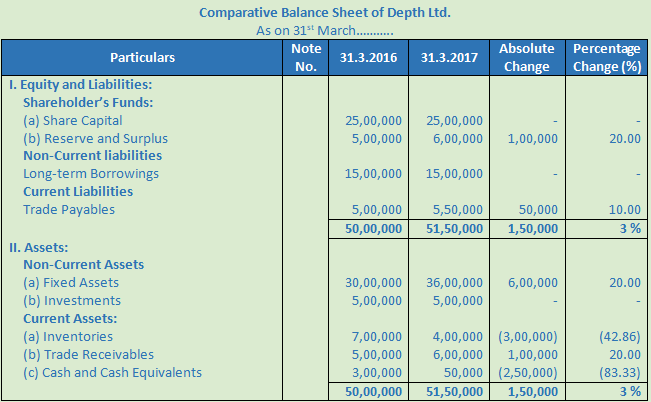Question 18. Prepare a Comparative Statement of Profit and Loss with the help of following information: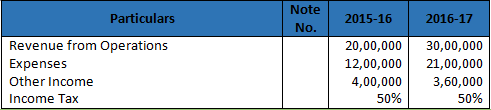Solution 18.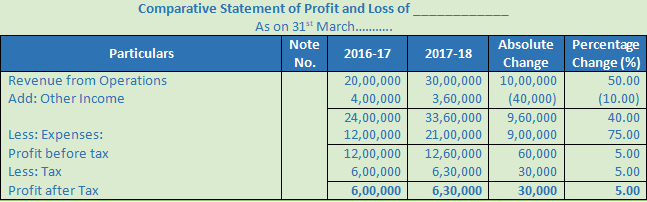Question 19. From the following information, prepare a Comparative Statement of Profit and Loss: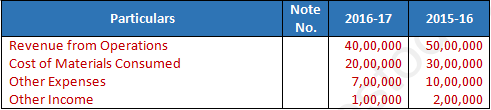Solution 19.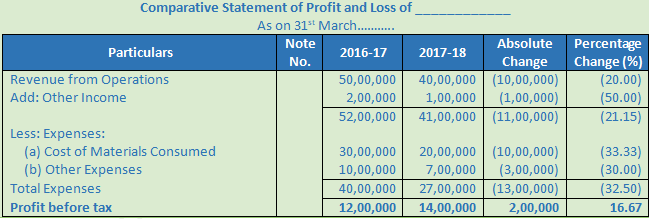Question 20. Prepare a Comparative Statement of Profit and Loss from the following information:Solution 20.Question 21. Prepare a Comparative Statement of Profit from the following information: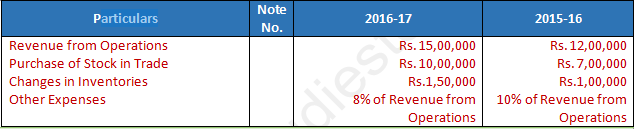Solution 21.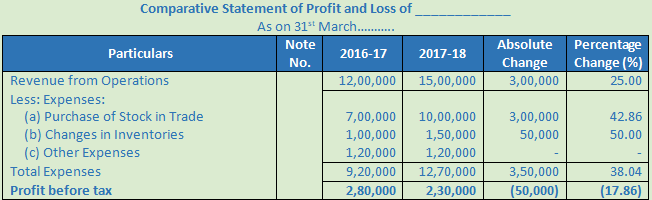Question 22. Prepare a comparative Statement of Profit of Varun Ltd. with the help of the following information:Solution 22.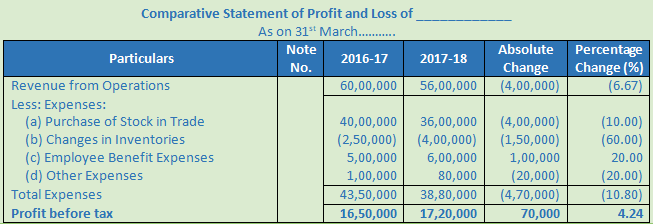Question 23. From the following data, prepare a Statement of Profit in the comparative form:Solution 23.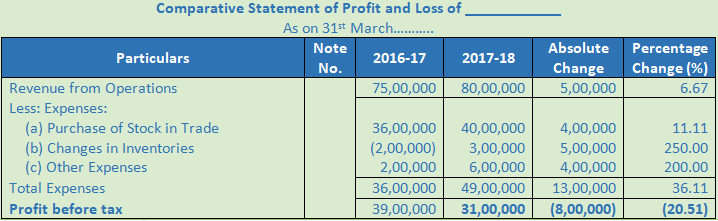Question 24. Prepare a Comparative Statement of Profit with the help of the following information:Solution 24.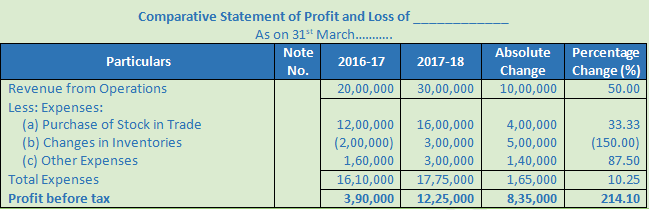Question 25. Profit and Loss Statements for the years ended 31st March 2017 and 2016:Calculate percentage changes from 2016 and 2017.

Solution 25.Question 26. Prepare a Comparative Statement of Profit and Loss from the following: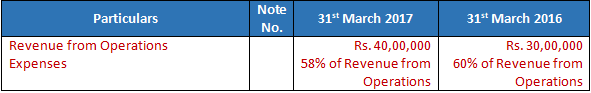Interest on investments @ Rs. 2,00,000 and taxes payable @ 50%.

Solution 26.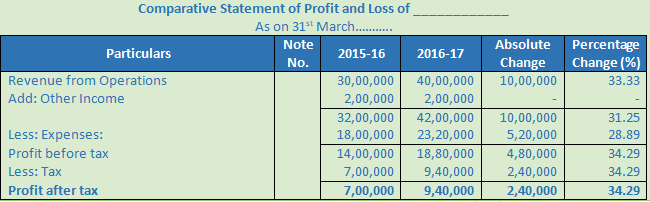Question 27. From the following information, prepare a Comparative Statement of Profit and Loss Zee Ltd:Solution 27.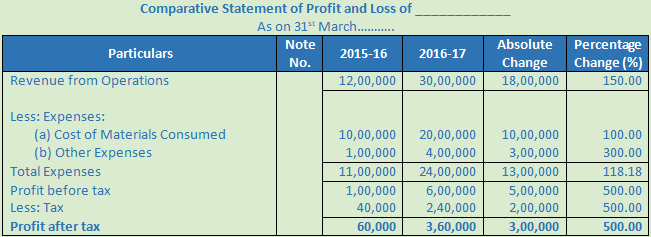Question 28. Prepare a Comparative Statement of Profit and Loss from the following information: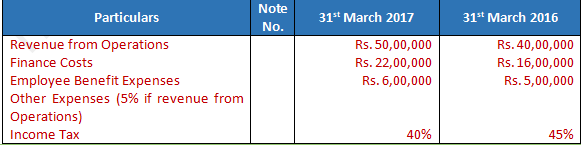Solution 28.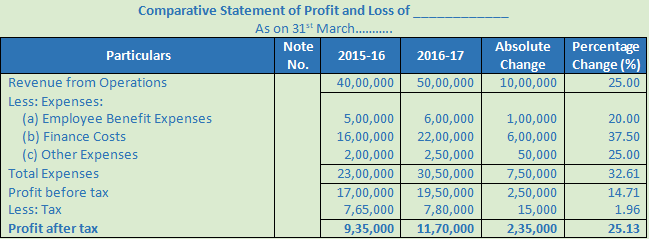Question 29. From the following Statement of Profit and Loss of Moontrack Ltd., for the years ended 31st March, 2011 and 2012, prepare a ‘Comparative Statement of Profit and Loss.’Solution 29.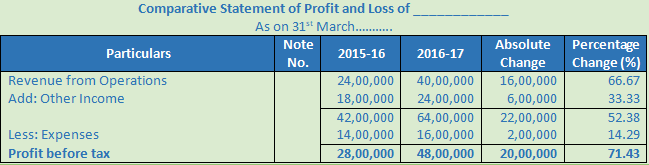Question 30. From the following Statement of Profit and Loss of Star Ltd., for the years ended 31st March, 2018 and 2017, prepare a ‘Comparative Statement of Profit and Loss.’Solution 30.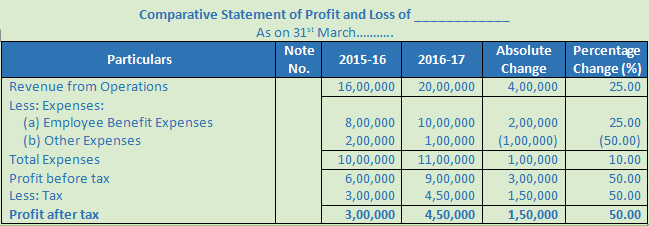Question 31. From the following ‘Statement of Profit and Loss’ of Fenox Ltd., for the years ended 31st March, 2018 and 2017, prepare a ‘Comparative Statement of Profit and Loss.’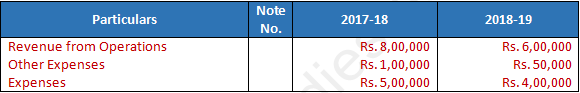Rate of income tax was 40%.

Solution 31.